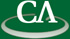## Computing Reliability Polynomials

### Mike Monagan, CECM, SFU

Wednesday September 28th, 2011 at 3:30pm in K9509.

```Abstract:

The reliability polynomial R(G,p) of a network G is a polynomial in p that
is the probability that the network G remains connected when each edge in G
may "fail" with probability p.  It is thus a theoretical measure of the
reliability of the network.  The reliability polynomial is related to the
Tutte polynomial from graph theory.  Like the Tutte polynomial, it is one of
many graph theoretic polynomials, such as the chromatic polynomial, which are
known to be NP-hard to compute.

In this talk I will first present the method based on edge deletion and edge
contraction for computing the reliablity polynomial.  I will then present
some Graph decompositions which facilitate the computation of R(G,p) and the
connection with the Tutte polynomial.  I will present our implementation of
this method in Maple, and compare it with Jeff Farr's Maple 12 implementation
in the GraphTheory package.

```